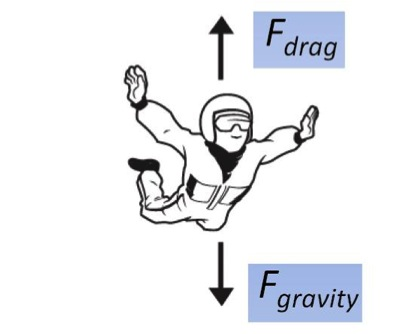# Skydiver

Calculus Level 1Two forces act on a parachutist. One is $mg,$ the attraction by the earth, where $m$ is the mass of the person plus equipment and $g=9.8 \text{ m/sec}^2$ is the acceleration of gravity. The other force is the air resistance ("drag"), which is assumed to be proportional to the square of the velocity $v(t)$.

Using Newton's second law of motion (mass $\times$ acceleration = net force applied), set up an ordinary differential equation for $v(t).$

Let $k$ denote the drag coefficient.

×